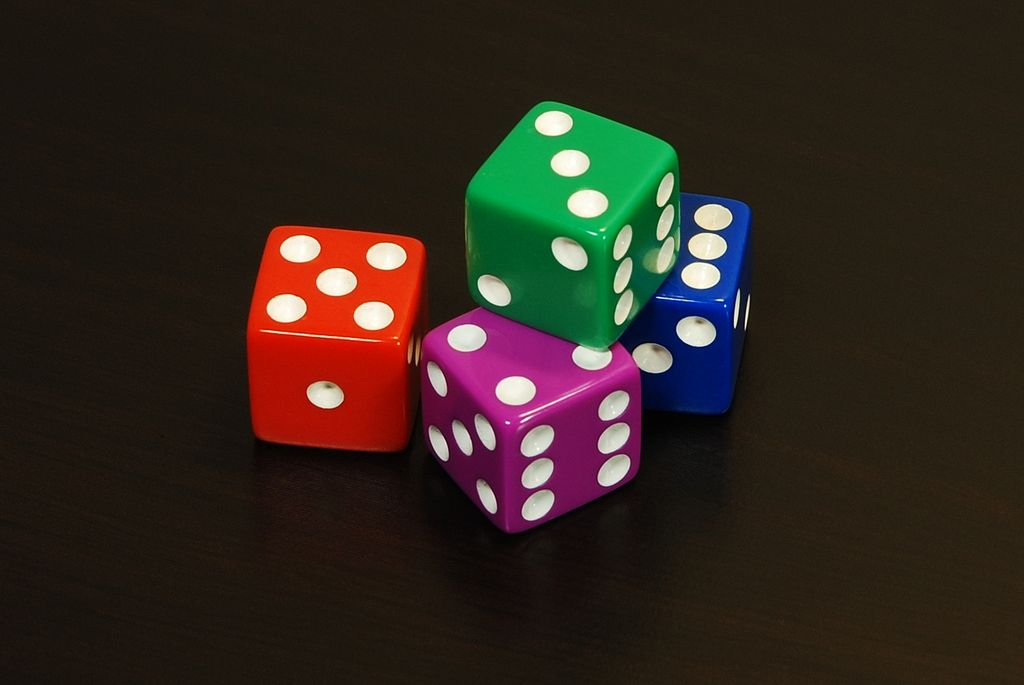# Even 4 dice can create havoc

Probability Level 5Four regular non-identical dice are rolled. The probability that the outcome is more than $14$ is of the form $\dfrac{A}{B}$, where $A$ and $B$

are co-prime integers.

Find the value of $B-A$.

$\textbf{Details and Assumptions}$

• Outcome means

$\displaystyle\sum_{n=1}^{4}(\text{number appearing on} \ n^{\text{th}} \ \text{die})$

• The four dice are regular ones, numbered $1, \ 2, \ \cdots , \ 6$.

• The four dice are not identical. You can consider them to be of four different colors.

×